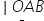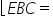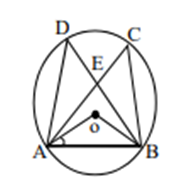Maths-
General
Easy

Question

# In the fig. ‘O’ is the centre of Circle. Chords AC and BD intersect at right angles at E, if= 35° then## 35°    45°    40°    50°Hint:

## The correct answer is: 35°

### A line is a simple, one-dimensional shape that can go on forever in opposing directions. A line may be vertical or horizontal. It can be drawn either top to bottom or left to right.When the ends of two rays collide at a single location, an angle is a geometry that results. They are expressed as radians or degrees (°). A 360-degree angle is the same as a whole rotation. It is symbolized by the character "∠".If a triangle side is created, the outside angle that results is equal to the product of the two opposite internal angles.Here we used the concept of angle sum property of a triangle. When two lines cross at one point, a linear pair of angles is created. If the angles follow the intersection of the two lines in a straight line, they are said to be linear. A linear pair's total angles are always equal to 180°. So here the angle EBC is 35 degree.#### With Turito Foundation.#### Get an Expert Advice From Turito.